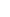DOPPLER TRIANGLEDoppler Triangle: They are very special triangles whose side lengths are determined by travel time of the signals.Doppler Triangles are used to analyze how (c+v)(c-v) mathematics takes place between two reference systems, one of which is in motion and the other is motionless. The positions of the reference systems at the moment the signal is emitted and the signal arrives form the vertexes of the triangle. EXPLANATION:In the figure above, we see a triangle which is formed by the points O, A and B. The Red line that is connected to the reference system of the plane is an auxiliary line that we will make use of while doing the analysis. d0 line: It is the incoming direction of the signal relative to the reference system of the observer. As the signal will cover this line at c speed, the travel time of the signal is tΔ=d0/c. d1 line: It is the distance that the plane covers at u speed within the travel time of the signal. d1=u.tΔ d2 line: It represents the outgoing direction of the signal relative to the reference system of the plane.  Red line: The red line that moves along with the plane shows the outgoing direction of the signal relative to the reference system of the plane. This line is parallel and equal in length to d2. Therefore, the Black d2 line represents the outgoing direction of the signal relative to the reference system of the plane.As we can see in the animation, the signal covers AO and BO distances in the same time. Because of d0=c.tΔ and d0<>d2, it should be d2= (c±v). tΔ. In other words, the signal covers d2 distance at (c±v) speed. "±v" value is the moving away/approaching speed of two reference systems.As can be seen, Doppler Triangle directly leads us to (c+v)(c-v) mathematics. Doppler Triangle is an indispensable part of (c+v)(c-v) mathematics. EXPLANATION:As can be seen in the figure, the observer sends the signal. d0 line: The Red line that moves together with the plane shows the incoming direction of the signal relative to the reference system of the plane. The signal covers the red line at c speed and reaches the plane. This line is parallel and equal in length to d0. Therefore, in the calculations, the black d0 is the line that determines the travel time of the signal.  d1 line: It is the distance that the plane covers at u speed within the travel time of the signal. d2 line: It is the direction of the outgoing signal according to the reference system of the observer and the signal covers this distance within the travel time. If we divide the d2 distance to travel time, we obtain the speed of the signal that is going towards the plane relative to the reference system of the signal tower. EXPLANATION:In this figure, the plane and the signal tower send signals to each other.INCOMING SIGNALS: Because the length of d0 (the red line for the plane) which determines the INCOMING direction of the signal does not change for the two reference systems and the signals cover d0 at c speed, the travel time is the same for both reference systems.  tΔ=d0/cOUTGOING SIGNALS: We see that the length of d2 (the blue line for the plane) which gives us the OUTGOING direction of the signal does not change for the two reference systems. As OUTGOING signals reach their targets at the same time and cover the same distance, the speeds of the signals that they send are equal for both reference systems.  c±v=d2/tΔ EXPLANATION:From two reference systems which are in motion relative to each other, we can assume that one of them is in motion and the other is motionless. In the animation above, in the figure on the left, the plane is in motion and the observer is motionless. In the figure on the right, it is assumed that the plane is motionless and the observer is in motion. We can see here that the same result will be obtained in both ways of thinking. When we press Play button, the animation shows us the equation.The plane is in motion; the observer is motionless. In the animation on the left, the red line that moves together with the plane is the INCOMING direction of the signal relative to the reference system of the plane. This line is parallel and equal in length to d0. Therefore, d0 is the line that determines the travel time of the signal. The time it takes for the signal to cover d0 is tΔ=d0/c. d2, on the other hand, is the outgoing direction of the signal relative to the reference system of the observer and the signal covers this line at c±v=d2/tΔ speed.The plain is motionless; the observer is in motion.  In the animation on the right, the travel time of the signal to the plane is again, as can be seen, determined by d0. The time it takes for the signal to cover d0 is tΔ=d0/c. The blue line connected to the Signal Tower is parallel and equal in length to d2 and gives us the distance that the signal going towards the plane covers relative to the reference system of the observer. Relative to the observer, the signal covers this line at c±v=d2/tΔ speed.Consequently, both ways of thinking give us the same result.v VALUE IN DOPPLER TRIANGLE Go back to the Animation List Page